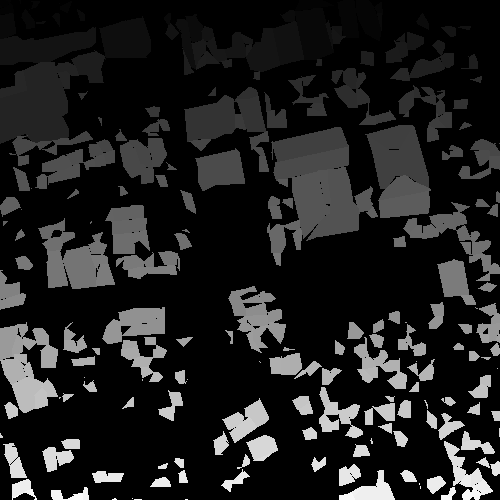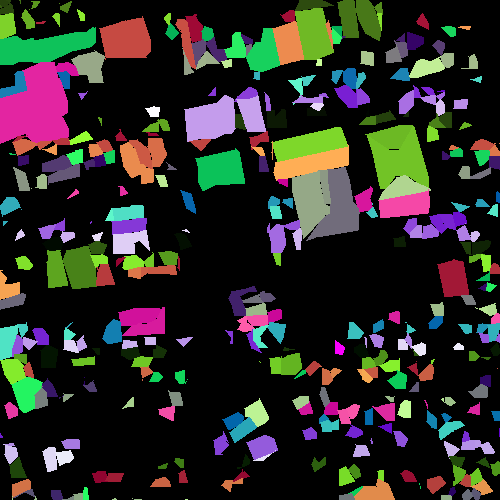# IndexedToRGBExample.cxx¶

Some algorithms produce an indexed image as output. In such images, each pixel is given a value according to the region number it belongs to. This value starting at 0 or 1 is usually an integer value. Often, such images are produced by segmentation or classification algorithms.

If such regions are easy to manipulate – it is easier and faster to compare two integers than a RGB value – it is different when it comes to displaying the results.

Here we present a convient way to convert such indexed image to a color image. In such conversion, it is important to ensure that neighboring regions, which are likely to have consecutive number have easily dicernable colors. This is done randomly using a hash function by `ScalarToRGBPixelFunctor`.The original indexed image (left) and the conversion to color image.

Example usage:

```./IndexedToRGBExample Input/buildingExtractionIndexed.tif Output/buildingExtractionRGB.png Output/buildingExtractionIndexed_scaled.png
```

Example source code (IndexedToRGBExample.cxx):

```#include "otbImage.h"
#include "otbImageFileWriter.h"
#include "itkUnaryFunctorImageFilter.h"
#include "itkScalarToRGBPixelFunctor.h"
#include "itkRescaleIntensityImageFilter.h"

int main(int argc, char* argv[])
{
if (argc != 4)
{
std::cerr << "Usage: " << argv << " <inputImageFile> ";
std::cerr << " <outputRGBImageFile> <outputScaledImageFile>" << std::endl;
return EXIT_FAILURE;
}
const char* inputFilename        = argv;
const char* outputRGBFilename    = argv;
const char* outputScaledFilename = argv;

using ImageType    = otb::Image<unsigned long, 2>;
using RGBImageType = otb::Image<itk::RGBPixel<unsigned char>, 2>;

// The UnaryFunctorImageFilter is the filter in charge of calling the functor
// we specify to do the work for each pixel. Here it is the ScalarToRGBPixelFunctor
using ColorMapFunctorType               = itk::Functor::ScalarToRGBPixelFunctor<unsigned long>;
using ColorMapFilterType                = itk::UnaryFunctorImageFilter<ImageType, RGBImageType, ColorMapFunctorType>;
ColorMapFilterType::Pointer colormapper = ColorMapFilterType::New();

using WriterType           = otb::ImageFileWriter<RGBImageType>;
WriterType::Pointer writer = WriterType::New();
writer->SetFileName(outputRGBFilename);
writer->SetInput(colormapper->GetOutput());

writer->Update();

// The following is just to produce the input image for the software guide
using OutputImageType          = otb::Image<unsigned char, 2>;